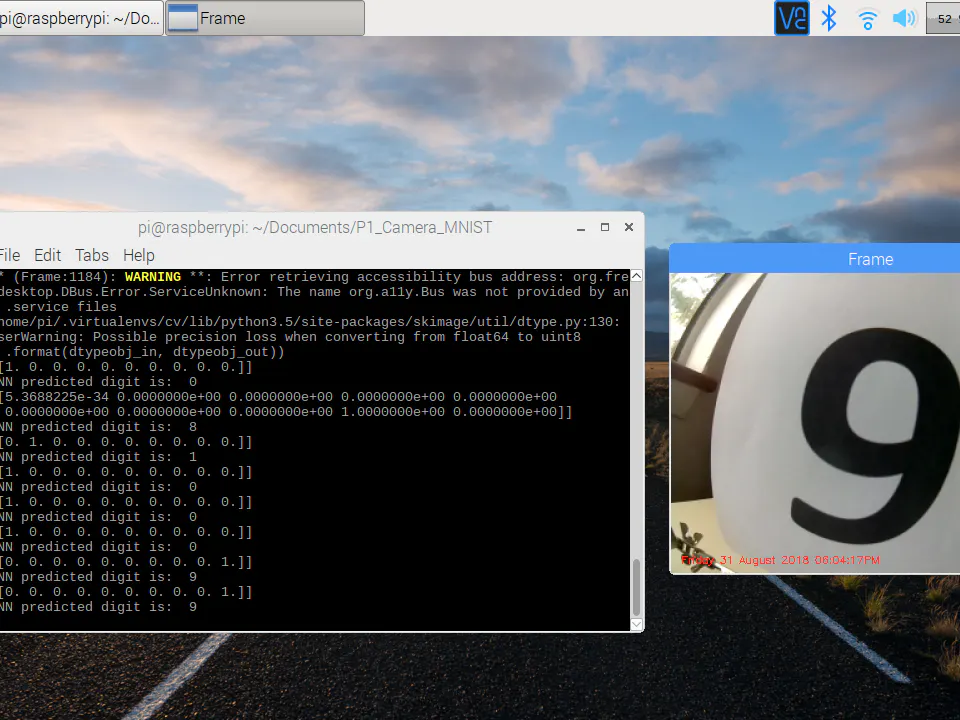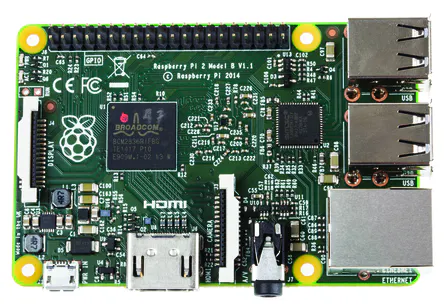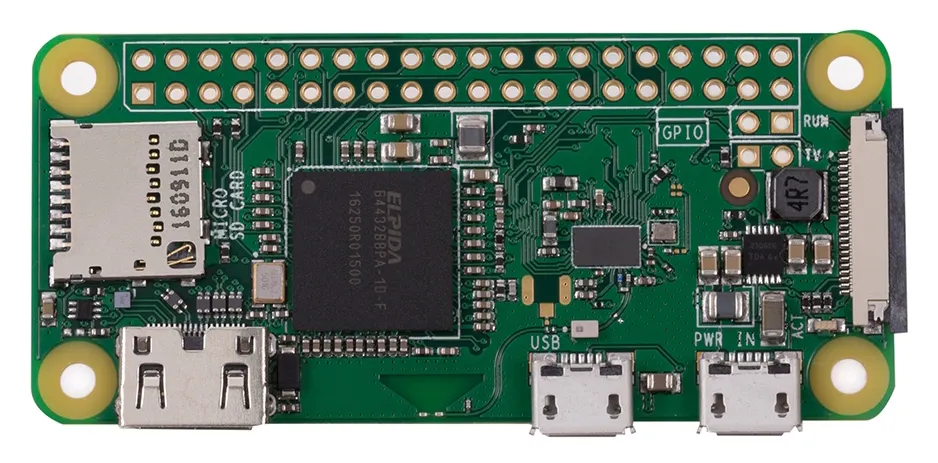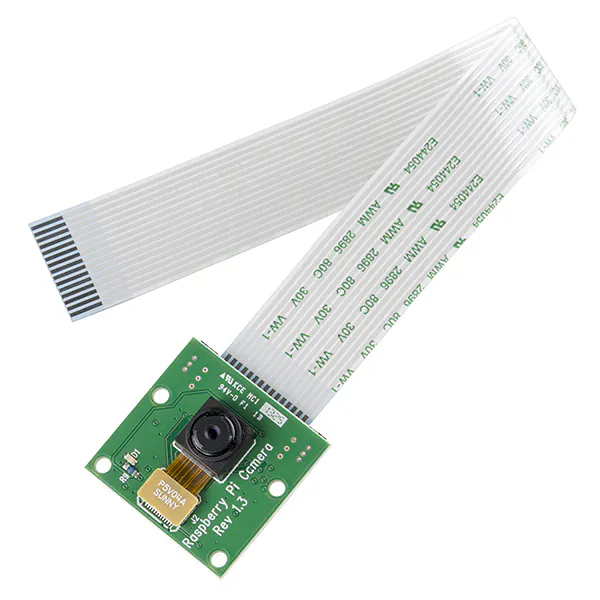AI Digit Recognition with PiCamera

Recognize digits with Raspberry Pi, Pi Camera, OpenCV, and TensorFlow.

BeginnerFull instructions provided20 hours18,427Things used in this project

Hardware componentsRaspberry Pi 3 Model B
×1Raspberry Pi 2 Model B
×1Raspberry Pi Zero Wireless
×1Raspberry Pi Camera Module
×1

Software apps and online servicesTensorFlowOpenCV

Schematics

PiCamera AI Digit Recogizer

Use Tensorflow and PiCamera to recognize digits

Code

Python
Digit recognition AI engine using Keras, Tensorflow , OpenCV and Raspberry PiCamera
#!/usr/bin/env python

# Copyright dhq 2018 Aug 31

#Theory of operation

# 2. convert to gray scale
# 3. convert to uint8 range
# 4. threshold via otsu method
# 5. resize image
# 6. invert image to balck background
# 7. Feed into trained neural network

#from skimage.transform import resize
import numpy as np
#from skimage import data, io
#from matplotlib import pyplot as plt
from skimage import img_as_ubyte		#convert float to uint8
from skimage.color import rgb2gray
import cv2
import datetime
import argparse
import imutils
import time
from time import sleep
from imutils.video import VideoStream

# construct the argument parse and parse the arguments
ap = argparse.ArgumentParser()
help="whether or not the Raspberry Pi camera should be used")
args = vars(ap.parse_args())

# initialize the video stream and allow the cammera sensor to warmup
vs = VideoStream(usePiCamera=args["picamera"] > 0).start()
time.sleep(2.0)

def ImagePreProcess(im_orig):
im_gray = rgb2gray(im_orig)				#convert original to gray image
#io.imshow(im_gray)
#plt.show()
img_gray_u8 = img_as_ubyte(im_gray)		# convert grey image to uint8
#cv2.imshow("Window", img_gray_u8)
#io.imshow(img_gray_u8)
#plt.show()
#Convert grayscale image to binary
(thresh, im_bw) = cv2.threshold(img_gray_u8, 128, 255, cv2.THRESH_BINARY | cv2.THRESH_OTSU)
#cv2.imshow("Window", im_bw)
#resize using opencv
img_resized = cv2.resize(im_bw,(28,28))
#cv2.imshow("Window", img_resized)
############################################################
#resize using sciikit
#im_resize = resize(im,(28,28), mode='constant')
#io.imshow(im_resize)
#plt.show()
#cv2.imshow("Window", im_resize)
##########################################################
#invert image
im_gray_invert = 255 - img_resized
#cv2.imshow("Window", im_gray_invert)
####################################
im_final = im_gray_invert.reshape(1,28,28,1)
# the below output is a array of possibility of respective digit
ans = model.predict(im_final)
print(ans)
# choose the digit with greatest possibility as predicted dight
ans = ans.tolist().index(max(ans.tolist()))
print('DNN predicted digit is: ',ans)

def main():
# loop over the frames from the video stream
while True:
try:
# grab the frame from the threaded video stream and resize it
# to have a maximum width of 400 pixels
frame = imutils.resize(frame, width=400)

# draw the timestamp on the frame
timestamp = datetime.datetime.now()
ts = timestamp.strftime("%A %d %B %Y %I:%M:%S%p")
cv2.putText(frame, ts, (10, frame.shape - 10), cv2.FONT_HERSHEY_SIMPLEX,
0.35, (0, 0, 255), 1)

# show the frame
cv2.imshow("Frame", frame)
key = cv2.waitKey(1) & 0xFF

# if the `q` key was pressed, break from the loop
if key == ord("q"):
break
# do a bit of cleanup
cv2.destroyAllWindows()
vs.stop()
elif key == ord("t"):
cv2.imwrite("num.jpg", frame)
ImagePreProcess(im_orig)
else:
pass

except KeyboardInterrupt:
# do a bit of cleanup
cv2.destroyAllWindows()
vs.stop()

if __name__=="__main__":
main()

Credits

Dimiter Kendri

21 projects • 129 followers
Working with AI, software, HDL, PCB design , robotics and electro-optics.
Thanks to Keras Team and Adrian Rosebrock.